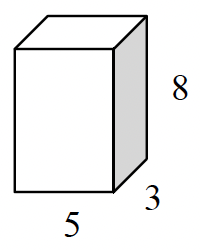### Home > GC > Chapter 9 > Lesson 9.2.1 > Problem9-59

9-59.

For a rectangular prism with base dimensions $3$ cm and $5$ cm and height $8$ cm:

1. Sketch the prism on your paper.2. Find the volume of the prism.

$\text{Volume of a prism} = \left(\text{area of the base}\right)\left(\text{height}\right)$

$120 \text{ cm}^3$

3. Find the surface area of the prism.

Find the area of each of the faces and then find their sum.

$158 \text{ cm}^2$